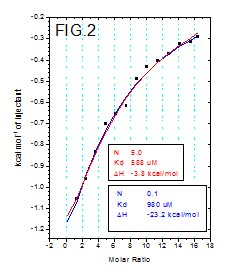The N-value commonly referred as the stoichiometry is often used as a qualifier for a “good” or “bad” titration. The reasoning goes like this – “if my N-value is 20% or more different from the expected stoichiometry (often 1) then my titration results are invalid”. In reality this is not always the case. It is important to understand that the ITC N–value is not the same thing as the stoichiometry (binding ratio). N-value does in fact equal the stoichiometry if the concentrations we use for the fitting are correct and 100% active. One way to express the N-value is the following:Any of the three values in this equation can change N, which means that N is a measure of the binding ratio as much as the activity of one’s samples. Two of these values have to be known (or assumed) in order to get the third one. For example if one is interested in the stoichiometry then the concentrations provided in the fit have to be accurate and 100% active. If one is interested in the activity of the sample in the cell (often protein), then the stoichiometry and the active concentration of the sample in the syringe (ligand) must be assumed.

Consider FIG.1:The provided concentrations are 20uM protein (in the cell) and 200uM ligand (in the syringe), N=0.86. Probably one of these two (or both) concentrations is not accurate, the likely binding ratio is either 1:1 (N=1) or 1:2 (N=0.5, 2 proteins: 1 ligand). If we assume it is 1:1 and the 200uM ligand is correct then refitting the data with 17uM protein will bring the N to 1, meaning that the actual binding competent concentration of the protein is 17uM. In the new PEAQ Data Analysis software any of the 3 unknowns (N, [protein],[ligand]) can be assigned as variable.

N-value can be easily visualized as the X-axis value at the mid (inflection) point of an ITC sigmoidal curve. There are certain titrations that do not have a clear inflection point – they are either with 1) low C-value (flat curve) or 2) reach saturation “too soon” either because the active concentration in the cell is too low or the ligand concentration is too high.

1)      In the case of low C value, the issue is weak affinity and low concentrations resulting in flat curve. Consider FIG.2:Kd is in the mid to high uM range but the protein concentration is only 50uM, this results in flat curve. In order to turn it into sigmoid with well-defined mid-point we need to have protein concentrations of 10x (or higher) above the Kd. Often this is not an option so researchers resort to the so called Low C value titration – in this case the protein concentration is kept low but the ligand concentration is increased so the curve reaches some degree of saturation (notice the high molar ratio). Such curve lacks inflection point so the N value is unknown and it has to be assumed and fixed to a constant value during the fit.

2)      In the case of the “early” saturation – the issue is inaccurate concentrations. The N value appears very small. For example on FIG.3:If we let the N vary during the fit we’ll get a value of 0.0008 which is certainly not the actual stoichiometry, moreover the dH is unusually high (dH for biological interactions rarely exceed 30 kcal/mol) .

How to extract reliable Kd and dH data from such titrations? By performing fits with couple of assumed extreme values of N and recording the Kd and dH variation. For example we can assume that the stoichiometry for FIG.2 is somewhere between 0.1 and 5 based on the reasonable quality of the fits we get with these 2 borderline scenarios. In this case the Kd varies very little – from 980uM to 588uM respectively. Similarly for fig3, the Kd varies from 25uM to 37uM for N from 0.2 to close to 0.

Even though non-sigmoidal curves are more challenging to analyze they should not be dismissed as inadequate results. Kd values may not be known with absolute certainty but they do usually converge to values within relatively narrow range even when the N-value (and by extension the active concentrations) uncertainty is large.

Related Resources:

Register for our upcoming webinar on advanced ITC data analysis with AFFINImeter: How to gain insights into complex modes of interaction with ITC

Playback the live launch of the new MicroCal PEAQ-ITC range